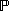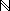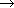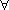### STATE

Based on chapter 5 in The Way of Z.

Links to more Z lectures.

This page looks best when thisand this X are about the same size:X. See these viewing tips.

A state is a situation or state of affairs''.

We model a state as an assignment of values to a collection of named variables.

States can represent assumptions (preconditions), goals (results, postconditions), or safety requirements (invariants).

State can be divided into fixed configuration (constants) and volatile components (state variables).

Programming languages do not describe states directly; they can only describe state transitions.

STATE TRANSITIONS

Programming languages describe state transitions.

  int iroot(int arg)
/* Integer square root */
{
int i, term, sum;

term = 1; sum = 1;
for (i = 0; sum <= arg; i++) {
term = term + 2;
sum = sum + term;
)
return i;
)


STATES

Specification languages describe states directly.iroot:a:iroot(a) * iroot(a)a < (iroot(a)+1) * (iroot(a)+1)Back to Z lectures.

Jonathan Jacky / University of Washington / Seattle, Washington / USA
E-mail: jon@u.washington.edu